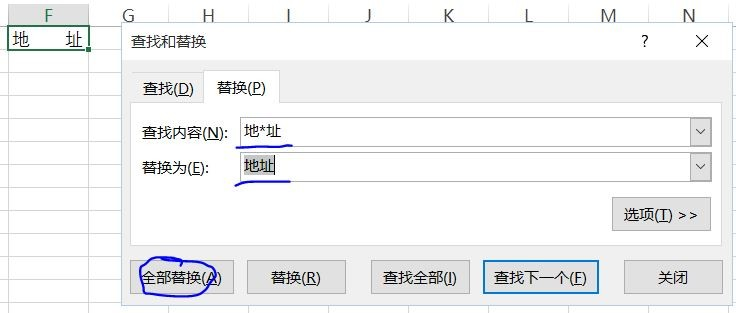• ZOL首页
• 产品报价
• 论坛
• ZOL问答
• 更多
• 手机客户端

• 回答数

6

• 浏览数

585

### 6个回答 默认排序 默认排序 按时间排序

“查找和替换”中，使用通配符*代替若干个空格。=IF(LEFT(A1,1)=" ",MID(A1,2,LEN(A1)),A1)

=IF(Sheet1!\$A1="",IF(Sheet1!B1="","",Sheet1!B1),IF(Sheet1!A1="","",Sheet1!A1))

Sheet2工作表就是你要的结果。

### 最新回答 更多>

ZOL问答 > 怎么批量去掉excel中两字间的空格?

### 举报成功